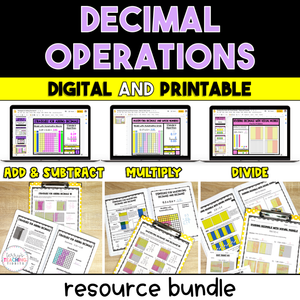# Decimal Operations Resource Bundle - Digital & Printable

• \$43.00
Unit price per
• Save \$14
Shipping calculated at checkout.

Use visual models along with the standard algorithm and area models to quickly and effectively teach your students how to add, subtract, multiply, and divide decimals with this comprehensive printable and digital resource pack.

With 11 mini-lessons, (2 adding and subtracting decimals, 3 multiplying decimals, and 6 dividing decimals - 5 using the standard algorithm and one with visual models) you will have plenty of resources to teach your student how to solve problems using decimal operations.

Using visual models, students will have a more hands-on approach to “see” what it looks like to add, subtract, and multiply decimals as well as divide decimals using tenths and hundredths.  These models will help reinforce the use and understanding of the standard algorithm.  It’s great for kinesthetic learners as well as students that may struggle with memorizing algorithms.

This resource is complete, comprehensive, and easy to follow with a clear format.  It is low-prep, easy to assign to a sub, and contains engaging printable activities and digital versions for use with Google Classroom.  If your students are struggling conceptually, this resource is for you!

Supports Common Core Standards (CCSS):  5.NBT.7

This resource is also included in a 5th Grade Math Year-Long Curriculum Bundle.

How Can You Use This?

Introduce each skill with the detailed set of notes and sample problems.  I suggest doing this in a whole group setting and displaying the PDF or pulling the slides up on a Smartboard if you’re in person or share the screen online if you’re teaching virtually.

Gradually release students to practice independently using the practice pages or slides activities.

When all skills have been taught, provide additional practice and review using the set of printable or digital task cards, then assess with the final quizzes when you feel students are ready.

WHAT OTHER TEACHERS ARE SAYING…

⭐️⭐️⭐️⭐️⭐️  This resource was AMAZING!!! It was so helpful in teaching students all about decimals. The visual models were awesome! Everything was thought out and so helpful in teaching these standards. Thank you so much!!   - Keri M.

⭐️⭐️⭐️⭐️⭐️  I LOVED how this was set up. There is a built in tutorial video, the manipulatives, all of it was so great. I can't recommend this enough.  - Jean B.

⭐️⭐️⭐️⭐️⭐️  I like that this resource has interactive slides for the students after using the notes and tutorials for instruction. The instruction videos being included in all aspects are great for the students to review independently when needed. There are a variety of questions and strategies presented, which is great for all types of learners.   - Kasey S.

## What You Get

Mini-Lessons Included:

• Subtracting Decimals
• Multiplying Decimals by Whole Numbers
• Multiplying Decimals by Decimals
• Multiplying Decimals Using Area Models
• Dividing Decimals by Whole Numbers
• Dividing Whole Numbers by Decimals
• Dividing Decimals by Decimals (with the same # of decimal places in the dividend and divisor)
• Dividing Decimals by Decimals (with a different # of decimal places in the dividend and divisor)
• Dividing Decimals by Decimals in which you have to annex a zero in the dividend
• Dividing Decimals by Decimals using a visual model

What You’ll Get in EACH of the mini-lessons:

• Detailed Notes
• Sample Problems
• Tutorial videos (digital versions only)
• Practice
• 2 Homework Pages (Dividing Decimals Printable Only)
• Exit Tickets (2+ *The amount varies depending on the mini-lesson*

You’ll Also Get:

• Task Cards (review) - Multiplying & Dividing Decimals Only